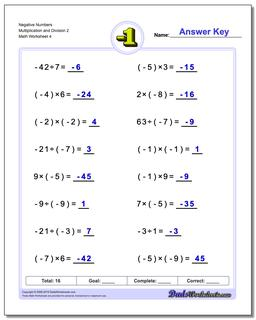One Dad. Four daughters. 9,251 worksheets... and counting!Negative Numbers Multiplication and Division 2 (Fourth Worksheet)

PropertyValue
DescriptionNegative Numbers Multiplication and Division 2: The worksheets in this section introduce negative numbers integers in multiplication and division math problems. All problems deal with smaller integers that can be solved without multi-digit multiplication or long division. (Fourth Worksheet)
Resource TypeWorksheet